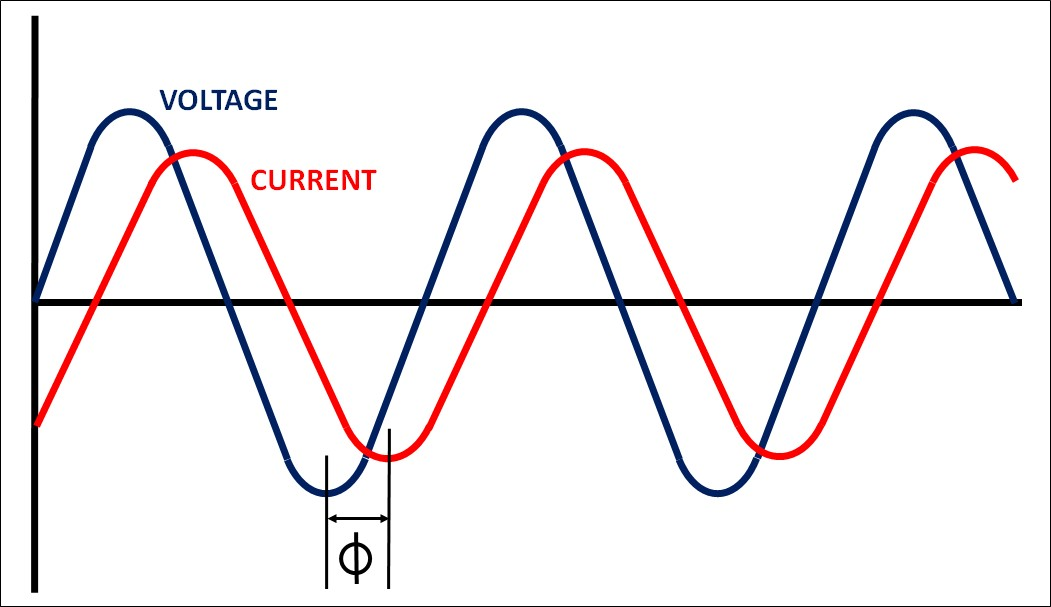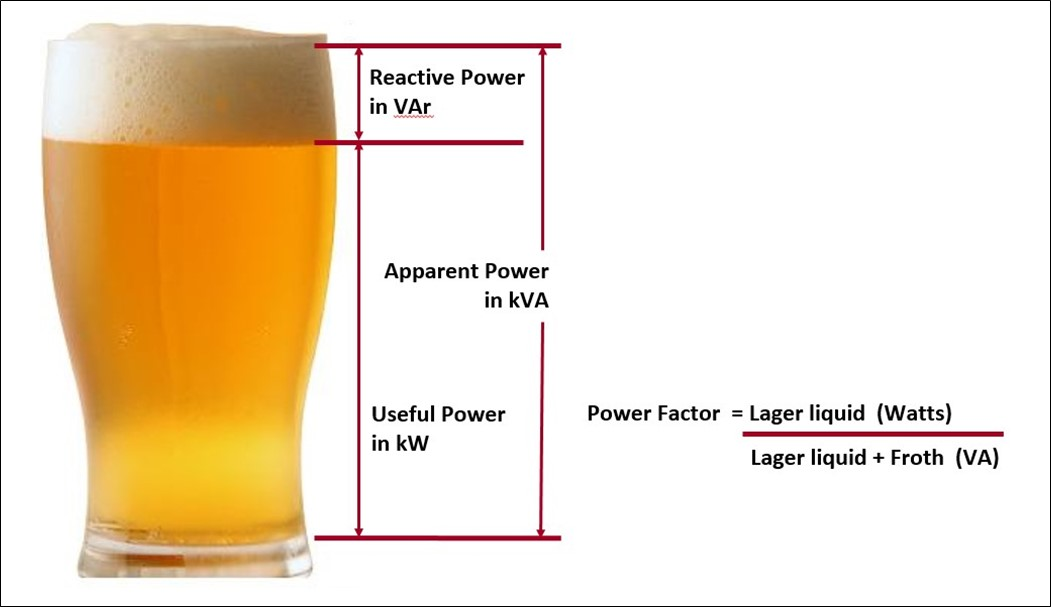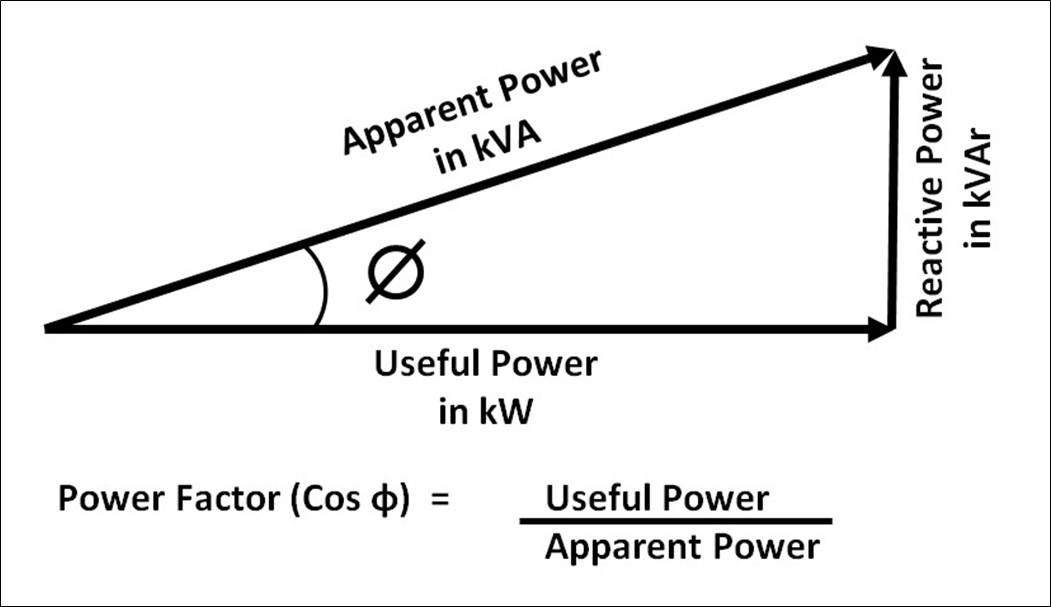The term LED stands for Light Emitting Diode, which is a semi-conductor in that it can conduct electrical current and when a current is applied across the LED, this results in the release of energy in the form of photons or light energy. The LED light source is low voltage but also relatively low current making it lower in power and significantly more efficient than the traditional incandescent lamp of previous times. The light power of a LED light is measured in Watts, which is by the equation of the electrical current measured in Amps multiplied by the supply voltage, measured in Volts.

Typically, an LED light source can be from less than a single watt to many watts and in the main, the greater the power in Watts, the greater the current in Amps and the greater the Lumen output. However, this is not always the case and this situation opens the concept of efficiency and that of Power Factor.

Power Factor is a way of describing how efficiently the electrical power is consumed and refers to the power within an alternating current (AC) electrical circuit, either for a single piece of equipment, such as a light fitting or for all of the electrical equipment being used on a complete application. There are two forms of Power Factor (PF) in an AC electrical system, that of Leading Power Factor where the current leads the voltage in the circuit, (relating to that of capacitive load) and Lagging Power Factor, the current lags the voltage in the circuit (relating to that of inductive load), as in the case of LED light sources.The power being used for the individual luminaire or complete circuit is drawn from the network and can be described as consisting of two parts, that of USEFUL POWER and REACTIVE POWER. The useful power (Real Power / True Power) is that which is required to undertake the task, such as that needed to provide illumination from a single light fitting and this is measured in watts, or more specifically kilowatts (kW). The reactive power is that used by a reactive load within the circuit but does not contribute to achieving the required task and can therefore be considered as being ‘wasted power’ and is measured in kilovolt-Amps Reactive (kVAR).

Taking the combination or the useful power required to illuminate the light fitting and the wasted reactive power, the resultant power is determined as the total power, referred to as the APPARENT POWER, which is measured in kilovolt-Amps (kVA). It should be noted that the total power is not the linear sum of the useful power and the reactive power.

In order to explain Power Factor in a more simplistic way, let’s consider it in respect of ordering a pint of lager at your local pub and the pint provided is as shown below. Let’s start with the froth which is determined as representing the Reactive Power measured in kVAr, whilst the liquid is determined as representing the Useful Power (Real Power / True Power) measured in kW and the total contents of the glass is determined as representing the Apparent Power measured in kVA. When ordering you pint of lager at the bar, what you want from it is more liquid, less froth, which is the same as in attributing this to a lighting fitting in that you want a luminaire with a higher proportion of Useful Power and a small amount of Reactive Power, which is a fitting with a higher Power Factor.Getting back to the technical aspect, Power Factor is defined as being the ratio between that of the useful power and that of the apparent power and is a dimensionless figure that is always less than or equal to 1.0. For resistive loads such as for incandescent lamps, the ratio between the useful power and that of the apparent power is generally unity and as such the Power Factor is 1.0, however for inductive loads, unity is not easily achieved and the apparent power is then higher than that of the useful power, resulting in a Power Factor (PF) value of less than 1.0.The lower the value of Power Factor, the less efficient the light fitting is in converting the apparent power into the useful power required to illuminate the fitting. These types of light fittings and lamps create more reactive power which uses up the power capacity within the system for no useful benefit.

In the case of a residential application where the power consumption is measured and billed in Kilowatt hours (kWHrs), there is no difference in the cost of the energy to the homeowner for differences in power factor, however, any lighting equipment which has a lower power factor will have a greater Apparent Power (VA) and when considering the electrical installation in respect of which size cable and circuit breakers to use for the lighting circuit, this Power Factor is to be considered and calculated appropriately by a qualified electrical contractor for each individual application as it may have an effect on the size and capacity of equipment being used for the installation.

For the many commercial customers such as in offices, industrial buildings, factories, warehouses, hospitals, schools, universities and the like, the billing cost of consumed energy is based upon two components, that of ‘demand’ and ‘usage’. The demand portion is how much energy is required at any one time for the operation of the building or is the peak number of Kilowatts (kW) consumed during a short interval within the billing period, whereas the usage portion is how much energy is consumed as measured in Kilowatt hours (kWHrs) within the billing period. For a building which is not appropriately Power Factor corrected, any equipment which has a low power factor value , including that of the lighting equipment, will result in a higher value of ‘demand’ when the power is switched to operate the lighting equipment, which then may adversely affect that portion of the energy invoice by increasing the cost of the demand portion. The ‘usage’ part of the cost is the amount of energy (kWHrs) used over the billing period and the two components are added together to provide the cost to the customer.

In concluding, it is advisable to consider the Power Factor when specifying lighting fittings, whether it be for a residential installation or for a commercial project as lighting equipment with a lower Power Factor may require increased cables with a higher current rating and/or larger circuit breakers for the residential installation and for the commercial or industrial application, result in an increase in the energy usage and therefore the building’s operating costs.